Courses

# NCERT Solutions (Part - 2) - Structure of Atom Class 11 Notes | EduRev

## Chemistry Class 11

Created by: Mohit Rajpoot

## Class 11 : NCERT Solutions (Part - 2) - Structure of Atom Class 11 Notes | EduRev

The document NCERT Solutions (Part - 2) - Structure of Atom Class 11 Notes | EduRev is a part of the Class 11 Course Chemistry Class 11.
All you need of Class 11 at this link: Class 11

Ques 2.26: An atom of an element contains 29 electrons and 35 neutrons. Deduce
(i)  the number of protons and
(ii) the electronic configuration of the element.
Ans:-
(i) For an atom to be neutral, the number of protons is equal to the number of electrons.

∴ Number of protons in the atom of the given element = 29
(ii) The electronic configuration of the atom is

1s2 2s2 2p6 3s2 3p6 4s2 3d10.

Ques 2.27: Give the number of electrons in the species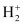, Hand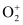Ans:-:

Number of electrons present in hydrogen molecule (H2) = 1 + 1 = 2

∴ Number of electrons in= 2 – 1 = 1

H2:

Number of electrons in H2 = 1 + 1 = 2:

Number of electrons present in oxygen molecule (O2) = 8 + 8 = 16

∴ Number of electrons in= 16 – 1 = 15

Ques 2.28: (i) An atomic orbital has = 3. What are the possible values of l and ml ?

(ii) List the quantum numbers (ml and l) of electrons for 3d orbital.

(iii) Which of the following orbitals are possible?

1p, 2s, 2p and 3f
Ans:-
(i) n = 3 (Given)

For a given value of n, can have values from 0 to (n1).

∴ For n = 3

l = 0, 1, 2

For a given value of l, ml can have (2l  1) values.

For l = 0, m = 0

l = 1, m = – 1, 0, 1

l = 2, m = – 2, – 1, 0, 1, 2

∴ For n = 3

l = 0, 1, 2

m0 = 0

m1 = – 1, 0, 1

m2 = – 2, – 1, 0, 1, 2
(ii) For 3d orbital, l = 2.

For a given value of l, mlcan have (2l  1) values i.e., 5 values.

∴ For = 2

m2 = – 2, – 1, 0, 1, 2
(iii) Among the given orbitals only 2s and 2p are possible. 1p and 3cannot exist.

For p-orbital, l = 1.

For a given value of n, can have values from zero to (n – 1).

∴ For l is equal to 1, the minimum value of n is 2.

Similarly,

For f-orbital, = 4.

For l = 4, the minimum value of is 5.

Hence, 1and 3f do not exist.

Ques 2.29: Using s, p, d notations, describe the orbital with the following quantum numbers.
(a) n = 1, = 0,
(b) n = 3; l =1,
(c) = 4; l = 2,
(d) n = 4; l =3.
Ans:- (a) n = 1, l = 0 (Given)

The orbital is 1s.

(b) For n = 3 and l = 1

The orbital is 3p.

(c) For n = 4 and l = 2

The orbital is 4d.

(d) For n = 4 and l = 3

The orbital is 4f.

Ques 2.30: Explain, giving reasons, which of the following sets of quantum numbers are not possible.
(a)   n = 0,  l = 0, ml = 0,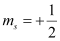(b)  n = 1,   l = 0,   ml = 0,
(c)   n = 1,  = 1,  ml = 0,(d)  n = 2, l = 1,  ml = 0,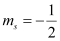(e)  = 3,   = 3,   ml = – 3,(f)  = 3,  = 1,  ml = 0,Ans:-
(a) The given set of quantum numbers is not possible because the value of the principal quantum number (n) cannot be zero.
(b) The given set of quantum numbers is possible.
(c) The given set of quantum numbers is not possible.

For a given value of n, ‘l’ can have values from zero to (n – 1).

For n = 1, l = 0 and not 1.
(d) The given set of quantum numbers is possible.
(e) The given set of quantum numbers is not possible.

For n = 3,

l = 0 to (3 – 1)

l = 0 to 2 i.e., 0, 1, 2
(f) The given set of quantum numbers is possible.

Ques 2.31: How many electrons in an atom may have the following quantum numbers?
(a) n = 4,(b) n = 3, l = 0
Ans:-
(a) Total number of electrons in an atom for a value of n = 2n2

∴ For n = 4,

Total number of electrons = 2 (4)2 = 32

The given element has a fully filled orbital as

1s2 2s2 2p6 3s2 3p6 4s2 3d10.

Hence, all the electrons are paired.

∴ Number of electrons (having n = 4 and) = 16
(b) = 3, l = 0 indicates that the electrons are present in the 3orbital. Therefore, the number of electrons having n = 3 and l = 0 is 2.

Ques 2.32: Show that the circumference of the Bohr orbit for the hydrogen atom is an integral multiple of the de Broglie wavelength associated with the electron revolving around the orbit.
Ans:- Since a hydrogen atom has only one electron, according to Bohr’s postulate, the angular momentum of that electron is given by: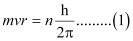Where,  = 1, 2, 3, …

According to de Broglie’s equation: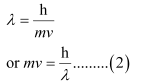Substituting the value of ‘mv’ from expression (2) in expression (1):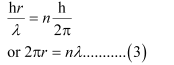Since ‘2πr’ represents the circumference of the Bohr orbit (r), it is proved by equation (3) that the circumference of the Bohr orbit of the hydrogen atom is an integral multiple of de Broglie’s wavelength associated with the electron revolving around the orbit.

Ques 2.33: What transition in the hydrogen spectrum would have the same wavelength as the Balmer transition n = 4 to n = 2 of He  spectrum?
Ans:-  For He+ ion, the wave number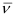associated with the Balmer transition, n = 4 to = 2 is given by: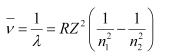Where,

n1 = 2, n2 = 4

Z = atomic number of helium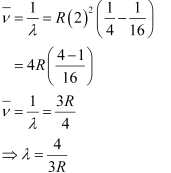According to the question, the desired transition for hydrogen will have the same wavelength as that of He+ .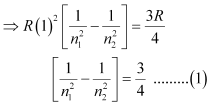By hit and trail method, the equality given by equation (1) is true only when n1 = 1and n2 = 2.

∴ The transition for n2 = 2 to n = 1 in hydrogen spectrum would have the same wavelength as Balmer transition n = 4 to = 2 of He+  spectrum.

Ques 2.34: Calculate the energy required for the process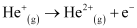The ionization energy for the H atom in the ground state is 2.18 ×10–18 J atom–1
Ans:- Energy associated with hydrogen-like species is given by,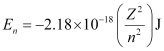For the given process,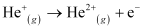An electron is removed from n = 1 to n = ∞.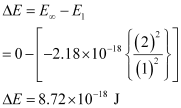∴ The energy required for the process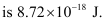Ques 2.35: If the diameter of a carbon atom is 0.15 nm, calculate the number of carbon atoms which can be placed side by side in a straight line across length of scale of length 20 cm long.
Ans:- 1 m = 100 cm

1 cm = 10–2 m

Length of the scale = 20 cm

= 20 × 10–2 m

Diameter of a carbon atom = 0.15 nm

= 0.15 × 10–9 m

One carbon atom occupies 0.15 × 10–9 m.

∴ Number of carbon atoms that can be placed in a straight line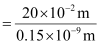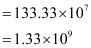Question 2.36: 2 × 108 atoms of carbon are arranged side by side. Calculate the radius of carbon atom if the length of this arrangement is 2.4 cm.

Ans:- Length of the given arrangement = 2.4 cm

Number of carbon atoms present = 2 × 108

∴ Diameter of carbon atom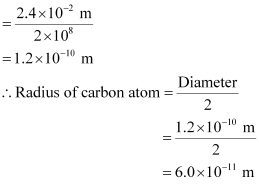Question 2.37: The diameter of zinc atom is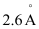.Calculate (a) radius of zinc atom in pm and (b) number of atoms present in a length of 1.6 cm if the zinc atoms are arranged side by side lengthwise.

Ans:-  (a) Radius of zinc atom = diameter/2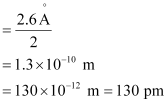(b) Length of the arrangement = 1.6 cm

= 1.6 × 10–2 m

Diameter of zinc atom = 2.6 × 10–10 m

∴ Number of zinc atoms present in the arrangement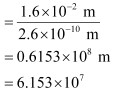Question 2.38: A certain particle carries 2.5 × 10–16C of static electric charge. Calculate the number of electrons present in it.

Ans:- Charge on one electron = 1.6022 × 10–19 C

⇒ 1.6022 × 10–19C charge is carried by 1 electron.

∴ Number of electrons carrying a charge of 2.5 × 10–16 C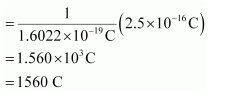Question 2.39: In Milikan’s experiment, static electric charge on the oil drops has been obtained by shining X-rays. If the static electric charge on the oil drop is –1.282 × 10–18C, calculate the number of electrons present on it.

Ans:- Charge on the oil drop = 1.282 ×10–18C

Charge on one electron = 1.6022 × 10–19C

∴Number of electrons present on the oil drop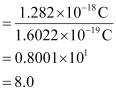Question 2.40: In Rutherford’s experiment, generally the thin foil of heavy atoms, like gold, platinum etc. have been used to be bombarded by the α-particles. If the thin foil of light atoms like aluminium etc. is used, what difference would be observed from the above results?

Ans:- A thin foil of lighter atoms will not give the same results as given with the foil of heavier atoms. Lighter atoms would be able to carry very little positive charge. Hence, they will not cause enough deflection of α-particles (positively charged).

Question 2.41: Symbols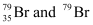can be written, whereas symbols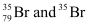are not acceptable. Answer briefly.

Ans:- The general convention of representing an element along with its atomic mass (A) and atomic number (Z) is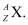Hence,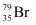is acceptable but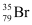is not acceptable.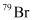can be written but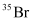cannot be written because the atomic number of an element is constant, but the atomic mass of an element depends upon the relative abundance of its isotopes. Hence, it is necessary to mention the atomic mass of an element

Question 2.42: An element with mass number 81 contains 31.7% more neutrons as compared to protons. Assign the atomic symbol.

Ans:- Let the number of protons in the element be x.

∴ Number of neutrons in the element

x  + 31.7% of x

= x + 0.317 x

= 1.317 x

According to the question,

Mass number of the element = 81

∴ (Number of protons number of neutrons) = 81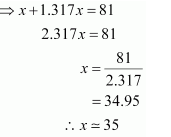Hence, the number of protons in the element i.e., x is 35.

Since the atomic number of an atom is defined as the number of protons present in its nucleus, the atomic number of the given element is 35.

∴ The atomic symbol of the element is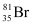.

Question 2.43: An ion with mass number 37 possesses one unit of negative charge. If the ion contains 11.1% more neutrons than the electrons, find the symbol of the ion.

Ans:- Let the number of electrons in the ion carrying a negative charge be x.

Then, Number of neutrons present =  + 11.1% of x

x + 0.111 x

= 1.111 x

Number of electrons in the neutral atom = (x – 1)

(When an ion carries a negative charge, it carries an extra electron)

∴ Number of protons in the neutral atom = x – 1

Given, Mass number of the ion = 37

∴ (x – 1) + 1.111x = 37

2.111x = 38

x = 18

∴The symbol of the ion is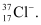Question 2.44: An ion with mass number 56 contains 3 units of positive charge and 30.4% more neutrons than electrons. Assign the symbol to this ion.

Ans:- Let the number of electrons present in ion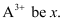∴ Number of neutrons in it = x + 30.4% of = 1.304 x

Since the ion is tri-positive,

⇒ Number of electrons in neutral atom = x  + 3

∴ Number of protons in neutral atom = x  + 3

Given, Mass number of the ion = 56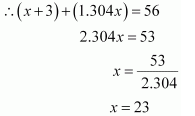∴ Number of protons = x + 3 = 23 + 3 = 26

∴ The symbol of the ion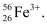Question 2.45: Arrange the following type of radiations in increasing order of frequency:

(b) amber light from traffic signal

(d) cosmic rays from outer space and

(e) X-rays.

Ans:- The increasing order of frequency is as follows:

Radiation from FM radio < amber light < radiation from microwave oven < X- rays < cosmic rays

The increasing order of wavelength is as follows:

Cosmic rays < X-rays < radiation from microwave ovens < amber light < radiation of FM radio

Question 2.46: Nitrogen laser produces a radiation at a wavelength of 337.1 nm. If the number of photons emitted is 5.6 × 1024, calculate the power of this laser.

Ans:- Power of laser = Energy with which it emits photons

Power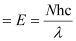Where,

N = number of photons emitted

h = Planck’s constant

Substituting the values in the given expression of Energy (E):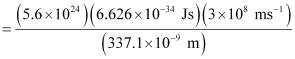= 0.3302 × 107 J

= 3.33 × 106 J

Hence, the power of the laser is 3.33 × 106 J.

Question 2.47: Neon gas is generally used in the sign boards. If it emits strongly at 616 nm, calculate

(a) the frequency of emission,
(b) distance traveled by this radiation in 30 s
(c) energy of quantum and
(d) number of quanta present if it produces 2 J of energy.

Ans:- Wavelength of radiation emitted = 616 nm

= 616 × 10–9 m (Given)

(a)  Frequency of emission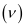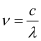Where,

Substituting the values in the given expression of: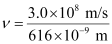= 4.87 × 108 × 109 × 10–3 s–1

ν = 4.87 × 1014 s–1

Frequency of emission (ν) = 4.87 × 1014 s–1

(b) Velocity of radiation, (c) = 3.0 × 108 ms–1

Distance travelled by this radiation in 30 s

= (3.0 × 108 ms–1) (30 s)

= 9.0 × 109 m

(c) Energy of quantum (E) = hν

(6.626 × 10–34 Js) (4.87 × 1014 s–1)

Energy of quantum (E) = 32.27 × 10–20 J

(d) Energy of one photon (quantum) = 32.27 × 10–20 J

Therefore, 32.27 × 10–20 J of energy is present in 1 quantum.

Number of quanta in 2 J of energy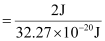= 6.19 ×1018

= 6.2 ×1018

Question 2.48: In astronomical observations, signals observed from the distant stars are generally weak. If the photon detector receives a total of 3.15 × 10–18 J from the radiations of 600 nm, calculate the number of photons received by the detector.

Ans:- From the expression of energy of one photon (E),

E = hc / λ

Where,

h = Planck’s constant

Substituting the values in the given expression of E:

E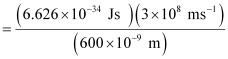E = 3.313 × 10–19 J

Energy of one photon = 3.313 × 10–19 J

Number of photons received with 3.15 × 10–18 J energy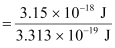= 9.5 ≈ 10

Question 2.49: Lifetimes of the molecules in the excited states are often measured by using pulsed radiation source of duration nearly in the nano second range. If the radiation source has the duration of 2 ns and the number of photons emitted during the pulse source is 2.5 × 1015, calculate the energy of the source.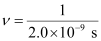ν = 5.0 × 108 s–1

Energy (E) of source = Nhν

Where,

N = number of photons emitted

h = Planck’s constant

Substituting the values in the given expression of (E):

E = (2.5 × 1015) (6.626 × 10–34 Js) (5.0 × 108 s–1)

E = 8.282 × 10–10 J

Hence, the energy of the source (E) is 8.282 × 10–10 J.

Question 2.50: The longest wavelength doublet absorption transition is observed at 589 and 589.6 nm. Calculate the frequency of each transition and energy difference between two excited states.

Ans:-

For λ1 = 589 nm

Frequency of transition

Frequency of transition (ν1) = c / λ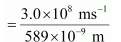= 5.093 × 1014 s–1

Similarly, for λ2 = 589.6 nm

Frequency of transition

Frequency of transition (ν2) = c / λ2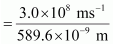=5.088 × 1014 s–1

Energy difference (ΔE) between excited states = E1 – E2

Where,

E2 = energy associated with λ2

E1 = energy associated with λ1

ΔE = hν1 – hν2

= h(ν1 – ν2)

= (6.626 × 10–34 Js) (5.093 × 1014 – 5.088 × 1014)s–1

= (6.626 × 10–34 J) (5.0 × 10–3 × 1014)

ΔE = 3.31 × 10–22 J.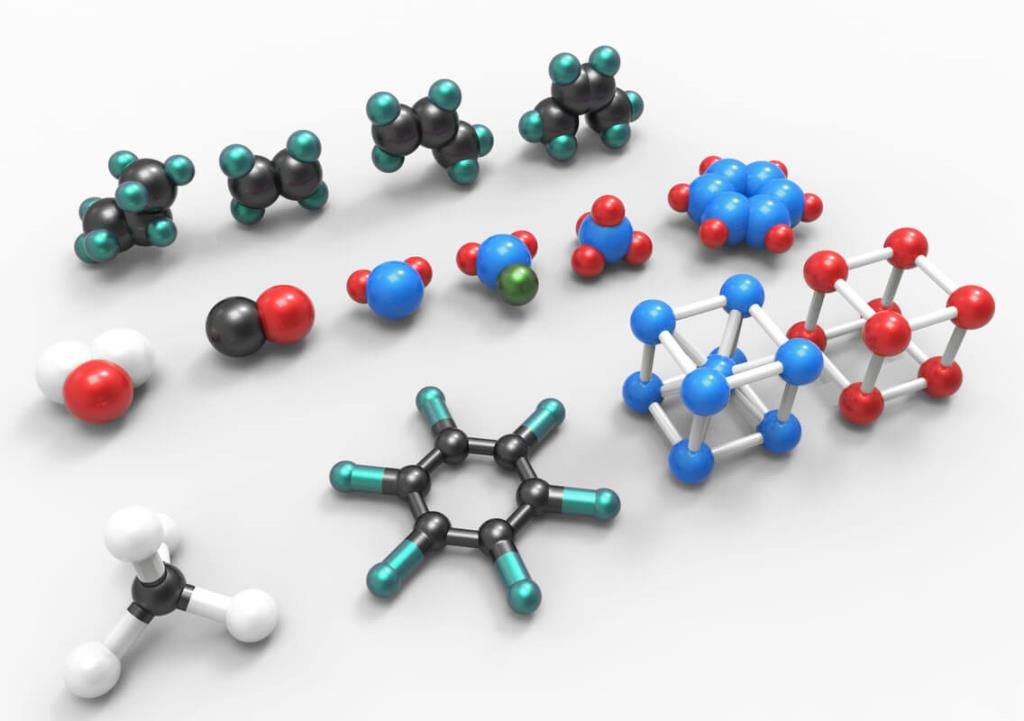Question 2.51: The work function for caesium atom is 1.9 eV. Calculate

(a) the threshold wavelength and

(b) the threshold frequency of the radiation. If the caesium element is irradiated with a wavelength 500 nm, calculate the kinetic energy and the velocity of the ejected photoelectron.

Ans:- It is given that the work function (W0) for caesium atom is 1.9 eV.

(a) From the expression,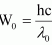, we get: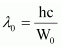Where,

λ0 = threshold wavelength

h = Planck’s constant

Substituting the values in the given expression of (λ0):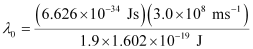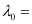6.53 × 10–7 m

Hence, the threshold wavelength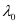is 653 nm.

(b) From the expression,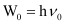, we get:

Vo = W0/h

Where, ν0 = threshold frequency

h = Planck’s constant

Substituting the values in the given expression of ν0: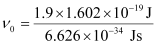(1 eV = 1.602 × 10–19 J)

ν0 = 4.593 × 1014 s–1

Hence, the threshold frequency of radiation (ν0) is 4.593 × 1014 s–1.

(c) According to the question:

Wavelength used in irradiation (λ) = 500 nm

Kinetic energy = h (ν – ν0)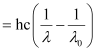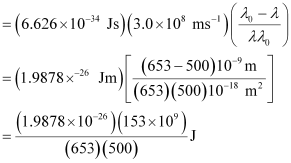= 9.3149 × 10–20 J

Kinetic energy of the ejected photoelectron = 9.3149 × 10–20J

Since K.E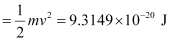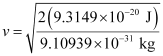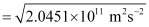= 4.52 × 105 ms–1

Hence, the velocity of the ejected photoelectron (v) is 4.52 × 105 ms–1.

Question 2.52: Following results are observed when sodium metal is irradiated with different wavelengths. Calculate (a) threshold wavelength and, (b) Planck’s constant.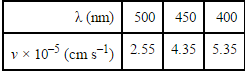Ans:-  (a) Assuming the threshold wavelength to be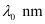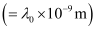, the kinetic energy of the radiation is given as: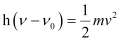Three different equalities can be formed by the given value as: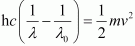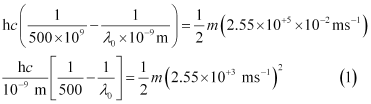Similarly,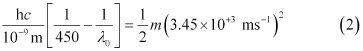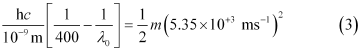Dividing equation (3) by equation (1):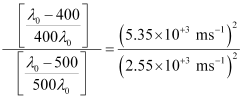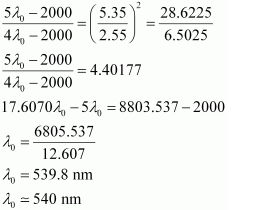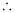Threshold wavelength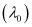= 540 nm

Note: part (b) of the question is not done due to the incorrect values of velocity given in the question.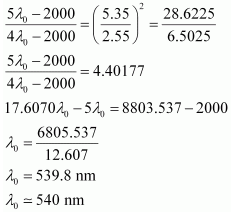,

,

,

,

,

,

,

,

,

,

,

,

,

,

,

,

,

,

,

,

,

;